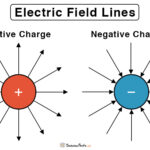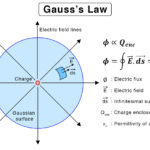Home / Physics / Lenz’s Law

# Lenz’s Law

We know that electromagnetic induction is governed by two fundamental laws – Faraday’s law and Lenz’s law. Faraday’s law establishes a relationship between the induced emf (ε) and the magnetic flux rate (dφ/dt) in a conducting coil of N turns.

Formula: ε = – N dφ/dt

However, the equation does not state anything about the conservation of energy. Lenz’s law can explain energy conservation and the negative sign in Faraday’s law equation.

## What is Lenz’s Law

Lenz’s law states that,

“The polarity of the induced emf is such that it opposes the change in magnetic flux that produced it.”

When a magnetic field induces a current in a conducting coil, the induced current generates its magnetic field, opposite to the inducing magnetic field. In other words, an induced current will always oppose the motion that started it in the first place. Lenz’s law is significant since it can determine the direction of the induced current and the magnetic field induced by the current.

The change in the magnetic flux around a conducting coil may be caused by several ways:

• Change the magnetic field strength
• Move the magnet toward or away from the coil
• Move the coil into or out of the magnetic field
• Rotate the coil relative to the magnet

Lenz’s law is named after German physicist Heinrich Friedrich Lenz after he deduced it in 1834.

## Lenz’s Law and Conservation of Energy

To obey the conservation of energy, the direction of the current induced via Lenz’s law must create a magnetic field that opposes the magnetic field that created it in the first place. The direction of this induced magnetic field is determined by the right-hand rule.

Suppose the current did not oppose the magnet’s magnetic field. Then, the induced magnetic field would be in the same direction as the inducing magnetic field. These two magnetic fields would add up and create a larger magnetic field. This larger magnetic field would induce another current in the coil twice the magnitude of the original current. This induced current will generate another magnetic field, and the process would continue. Thus, there would be an endless loop of induced currents and magnetic fields, violating the energy conservation law. Therefore, Lenz’s law is a consequence of the energy conservation principle.

## Applications of Lenz’s Law

Lenz’s law can be applied to the following devices:

• Inductor
• Electric generators
• Electromagnetic braking
• Induction cookware
• Eddy current equalizers
• Eddy current dynamometers
• Microphones

## Example Problems and Solutions

P.1. Calculate the magnitude of the induced emf when the magnet is thrust into a coil. The following information is given: the single loop coil has a radius of 5 cm, and the average value of the complex magnetic field component B cos θ increases from 0.1 T to 0.5 T in 0.2 s.

Soln. Given,

N = 1

r = 5 cm = 0.05 m

A = πr2 = π (0.05m)2 = 0.0079 m2

Δt = 0.2 s

(B cos θ)initial = 0.1 T

(B cos θ)final = 0.5 T

ΔB = (B cos θ)final – (B cos θ)initial = 0.5 T – 0.1 T = 0.4 T

|ε| = N Δφ/Δt

or, |ε| = N A Δ(B cos θ)/Δt

or, |ε| = 1 x 0.0079 m2 x 0.4 T/0.2 s = 0.016 Tm2/s = 16 mV

P.2. A circular coil of wire with 450 turns and a radius of 8 cm is placed horizontally on a table. A uniform magnetic field pointing directly into the wire and perpendicular to its surface is slowly turned on, such that the strength of the magnetic field can be expressed as a function of time as B(t) = 0.01(Ts-2) x t2. (A) What is the total emf in the coil as a function of time? (B) In which direction does the current flow?

Soln. Given,

B(t) = 0.01(Ts-2) x t2

N = 450

r = 8 cm = 0.08 m

A = πr2 = π(0.08 m)2 = 0.02 m2

ε = – N dφ/dt

or, ε = – 450 x d(BA)/dt

or, ε = – 450 x 0.02 m2 x d (0.01(Ts-2) x t2)/ dt

or, ε = – 0.09 x 2t Tm2/s2

or, ε = – 0.18t T/s

(B) The current will be clockwise looking from the top.

Article was last reviewed on Wednesday, September 1, 2021

### Related articlesElectric Field LinesToroid Magnetic FieldGauss’s LawElectric Flux